# How to calculate internal rate of return (plus definition and limits)Whether you’re an in-house accountant or function as an accountant consultant, you should be able to support with your clients with financial viability of projects. Usually, we evaluate projects using the Net Present Value (NPV) criteria.

According to Harvard Business Review, NPV is the present value of the cash flows at the required rate of return of your project, compared to your initial investment. It’s a simple way to calculate your return on investment. Another way to measure the financial success of a project is the internal rate of return (IRR). IRR is defined as a metric used to measure the profitability of an investment, that accounts for the time value of money.

In this article, we’ll share how to calculate internal rate of return and net present value, and how to navigate these calculations in Microsoft Excel. Follow along in the IRR worksheet of the workbook IRR.xlsx. This spreadsheet shows annual cash flows from two projects, as depicted in Figure 1.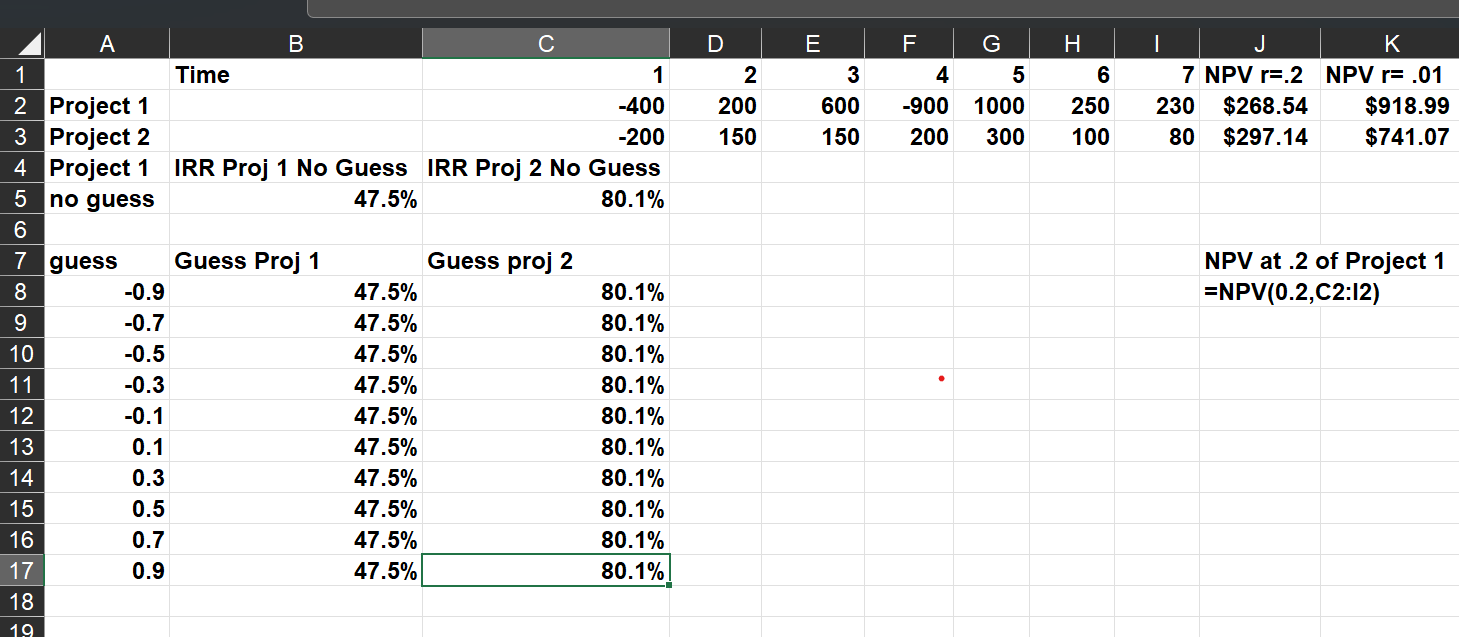Figure 1 Evaluation of two projects by NPV and IRR

## Calculating NPV in Excel

To utilize NPV a company assigns a discount rate to a project’s cash flows. A project with an NPV>0 helps the company. A project with an NPV<0 hurts the company.

The formula =NPV(0.2,C2:I2) in cell J2 computes the value of Project 1 in today’s dollars, using a 20% annual discount rate. We find an NPV of \$266.54, under the assumption that \$1 a year from now is calculated with formula =\$1/1.20.

Keep in mind: cells J1:K2 account for a discount rate of 20%. With this discount rate, Project 2 has a larger NPV. If we use a discount rate of 2%, Project 1 has a larger NPV. It’s often difficult to agree on a discount rate. This is when IRR becomes useful.

The IRR of a sequence of cash flows is the discount rate that makes the NPV of the cash flows equal to 0. There are several problems that can occur with IRR that we will discuss later, but in most situations, IRR tells you the rate of return you earn on your investment.

## How to calculate internal rate of return in Excel

The syntax of the IRR function is IRR(range of cash flows, [guess]). The guess argument is optional and should be a “guess” of what you think the IRR is. In most situations, you don’t need to enter the guess argument, but it is can sometimes be important.

To be on the safe side, you should enter a range of guesses. Copying from B8 to B9:B17 the formula =IRR(\$C\$2:\$I\$2,A8) shows us the IRR for Project 1 is 47.5%. Similarly, the formulas in C8:C17 show that for all guesses, our computed IRR is 80.1%. Essentially, this means that our investment in Project 1 earns an annual 47.5% rate of return, and our Investment in Project 2 earns an annual rate of return of 80.1%

## How to calculate internal rate of return with cash flows

How can we compute IRR when cash flows don’t occur at regular intervals? Excel’s amazing XIRR function comes to the rescue. Simply input the dates and values of the cash flows and Excel will return an investment’s annual rate of return. For example, in our worksheet, XIRR we find that the cash flows occurring on the displayed dates yield an annual IRR of 18.3%.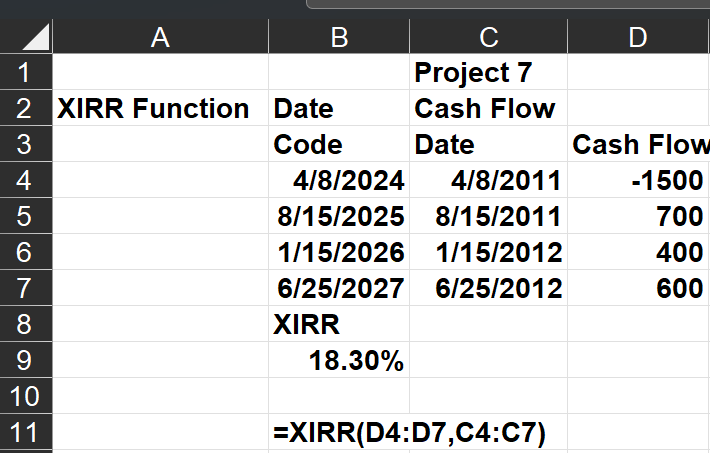Figure 2 An example of the XIRR function

## Does every investment have a unique IRR?

The three-period project shown in the No IRR worksheet of our spreadsheet has no IRR. This means there is no discount rate that will make the NPV of this project equal to 0.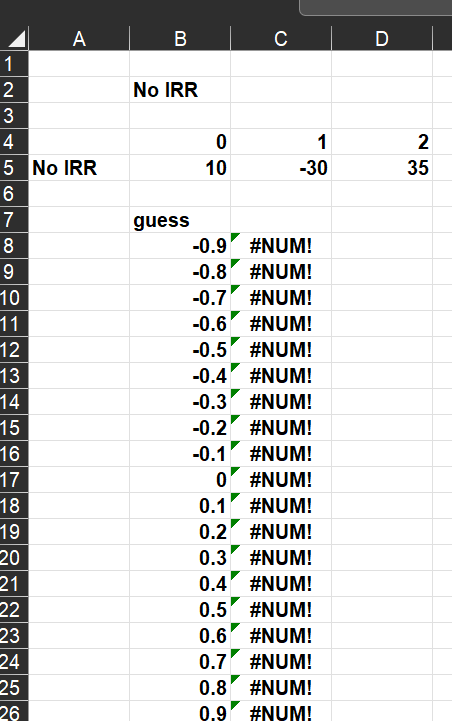Figure 3 A project with no IRR

As shown in the Multiple IRR worksheet, the project shown has two IRRs: -9.6% and 216.1%. We found their IRRs by varying our guess for the project’s IRRs. Both these discount rates yield an NPV of 0. There is no practical interpretation of IRR in this situation.

If a sequence of cash flow begins with a negative cash flow and is followed by all non-negative cash flows, then you are guaranteed a unique IRR.  Many projects meet this requirement because they start with a negative cash flow and then later cash flows are the positive returns on the investment.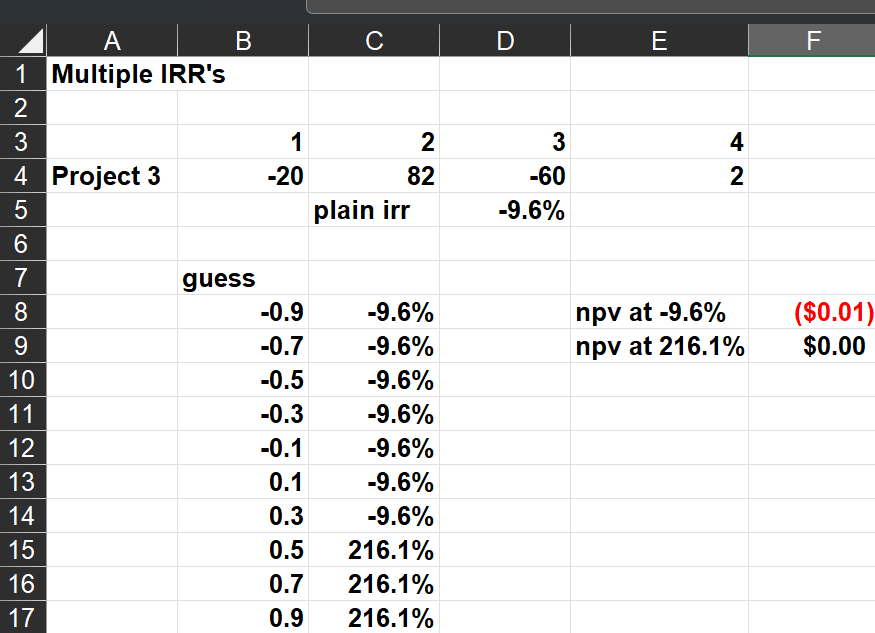Figure 4: A project with two IRR’s

## How are NPV and IRR related?

A sequence of cash flows has a positive NPV if and only if its IRR is greater than the discount rate. For example, a project with a 9% IRR will have a positive NPV for a discount rate less than 9% and will have a negative NPV for a discount rate of 9%.

## Should we always choose the project with the larger IRR?

Consider the two sequences of cash flows in the Which Project worksheet. Project 2 has an IRR of 50%, because \$1 grows in one year by 50%. Similarly, Project 1 has an IRR of 40%. If we were only allowed to choose one project, basing our decision on IRR would result in us choosing Project 2. This would be foolish, however, because Project 1 would put us in a much better Time 1 cash position than Project 2. This simple example shows that the IRR criterion may ignore the scale or size of the projects and yield to poor decision-making.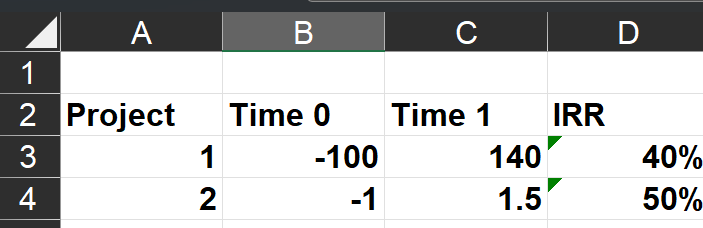Figure 5 Don’t choose the project with largest IRR!

IRR and NPV are essential functions in Microsoft Excel because they can help provide insight into financial forecasting for projects. Keep this resource handy and learn more Microsoft Excel functions for accountants with a CPE course.

You can learn more about Excel’s data analysis capabilities and earn CPE credit for free with a Becker CPE free demo. Just choose “Excel: Making Sense of Data 2023” while selecting your free webcast selection.

### Now Leaving Becker.com

You are leaving the Becker.com website. Once you click “continue,” you will be brought to a third-party website. Please be aware, the privacy policy may differ on the third-party website. Adtalem Global Education is not responsible for the security, contents and accuracy of any information provided on the third-party website. Note that the website may still be a third-party website even the format is similar to the Becker.com website.